I need help with automatic generated rows from Jet in Excel.

If i calculate 10 rows in table 2 in Jet and want to automatic copy it to table 1, excel shifts my rows in table 1 exactly with the automatic generated rows in table 2.

For example: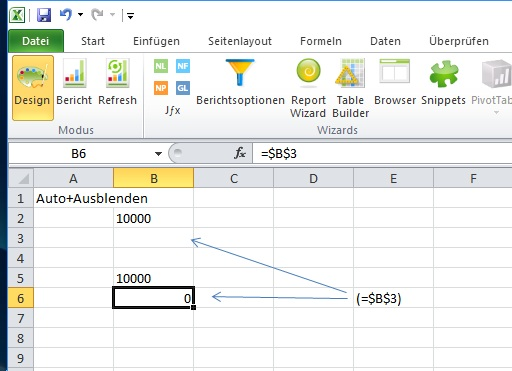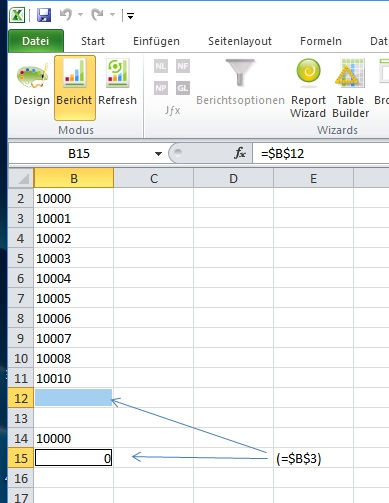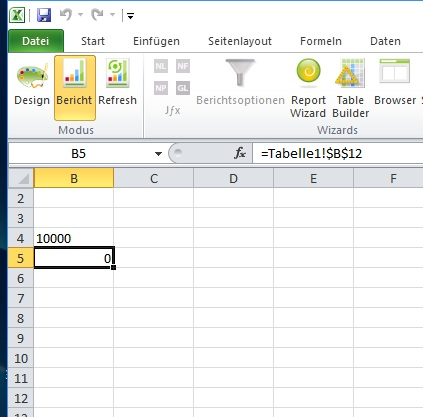formula (table 2; column A; row 1) automatic generated 10 results.

formula (table 1; column A; row 1 to row 10) = "Table2!\$A\$1"

After calculation excel shifts these formula in table 1 to = "Table2!\$A\$11"

How could it be solved?

Thanks for help!

#### 7 comments

•Harry Lewis

Hello David -

I assume that cell B2 contains a replicating function like this: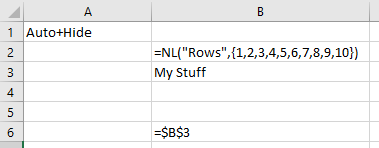When I run, I get these results: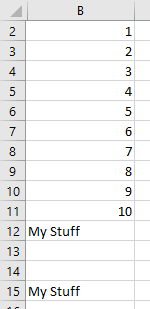What results are you trying to get?

•Nutzer

Dear Harry,

thanks for your response, my problem is a little bit tricky to declare.

I want to transfer the results of the replicating function.

For example, in the following table; B2 included my JetFunction =NL("Rows";......;"Limit=";10);

i want to bring the results from B2 to B13; all good; but i want to bring the results as follows; B13 = B2, B14 = B3, B15 = B4, ...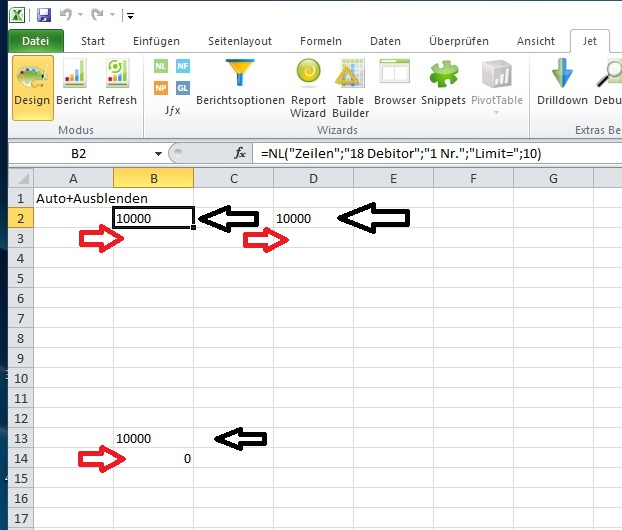i want to bring the results from B2 to B13; all good; but i want to bring the results as follows; B13 =B2, B14 =B3, B15 =B4, ...

Jet will bring my B13 =B2 to B22 =B2 as a follow of the function, all clear; but why Jet will dont do it with the following results.

Before Refresh: B13 =B2; B14 =B3; B15 =B4 (Thats what i want) and

it will dont help if i do it with \$B\$13 =\$B\$2; \$B\$14 =\$B\$3; \$B\$15 =\$B\$4

After refresh i got: B22 =B2; and here begins the problem B23 =B12 ??? WHY ??, how could it be solved.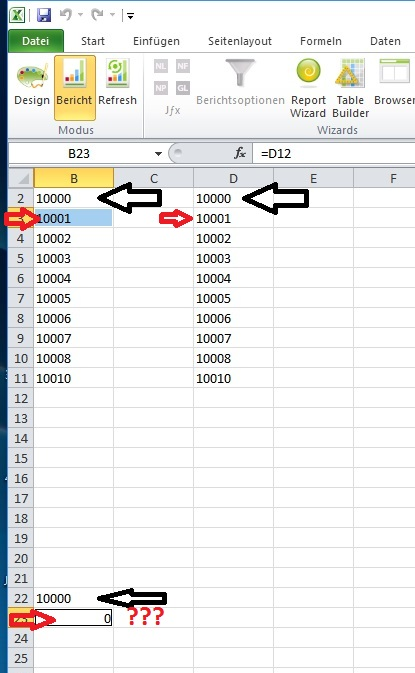Is there a solution, to got the results from the replication function to other rows without a problem?

Edited by Nutzer
•Harry Lewis

Hello David -

In order to get to that information, you would have to know how many results are being returned.  If your NL(Rows) function will always use the LIMIT= filter, you know already now that information.  If not, you would need to use NL(Count) to calculate it.

Here is one technique: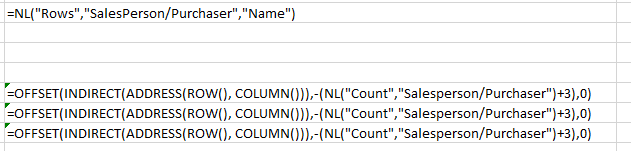which would produce results like this: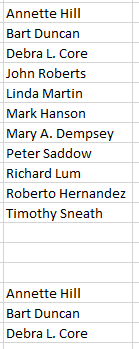•Heather Rowe

Is there any reason you cannot run the rows function again?

Once in row 2, and then again in row 5, so it would replicate down?

•Nutzer

Dear Harry,

thank you so much, it works with the Excel Offset function!

Edited by Nutzer
•Heather Rowe

never mind, I see you have a solution

Edited by Heather Rowe
•Nutzer

Dear Heather,

thanks for your comment. I found the solution with Harrys example.

The way is to work with the excel formula Offset; the beginning is the first row where the NL functions begin, for example D2;

the way to move the replicated rows to another sheet or table or column or row is to work with the Offset formula from Excel.

It helps me to become my wished results. The Offset-Formula, will begin with D2 but you can say bring me the result from Jets automatically moved cell to another one, it works only if you know where the results from Jet are inside.

Please sign in to leave a comment.